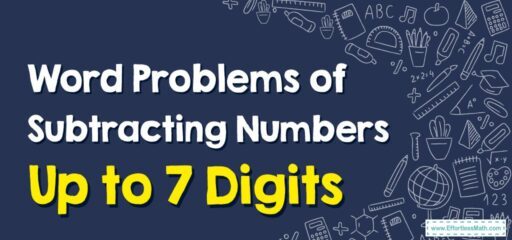# How to Solve Word Problems of Subtracting Numbers Up to 7 Digits

Word problems can be a bit tricky, but if you break them down into smaller steps, they become more manageable.## A Step-by-step Guide to Solving Word Problems of Subtracting Numbers Up to 7 Digits

Here’s a step-by-step guide on how to solve word problems involving subtraction with numbers up to 7 digits.

### Step 1: Read the problem carefully

The first step in solving any word problem is to understand what it is asking. Read the problem at least twice to make sure you understand it.

### Step 2: Identify the numbers and the operation:

Look for the numbers in the problem and identify the operation you need to perform. If it’s a subtraction problem, you’ll see words like ‘subtract’, ‘less’, ‘decrease’, ‘difference’, etc.

### Step 3: Write down the equation

Once you’ve identified the operation and the numbers, write down the equation. For example, if the problem is “John has 1,234,567 dollars. He spent 765,432 dollars. How much money does he have left?” the equation would be 1,234,567 – 765,432 = ?

### Step 4: Perform the subtraction

Line up the numbers by place value (ones, tens, hundreds, thousands, etc.), then start subtracting from the right (ones place) and move to the left. Remember to borrow from the next digit if the top number is smaller than the bottom number.

### What people say about "How to Solve Word Problems of Subtracting Numbers Up to 7 Digits - Effortless Math: We Help Students Learn to LOVE Mathematics"?

No one replied yet.

X
30% OFF

Limited time only!

Save Over 30%

SAVE $5 It was$16.99 now it is \$11.99